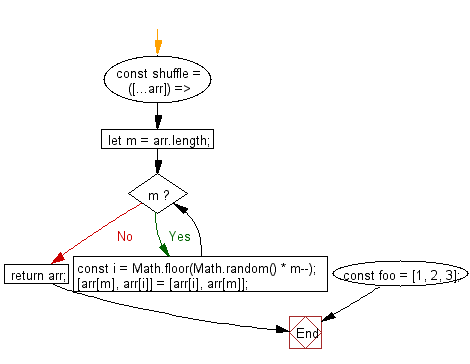# JavaScript: Randomize the order of the values of an array, returning a new array

## JavaScript fundamental (ES6 Syntax): Exercise-145 with Solution

Write a JavaScript program to randomize the order of array values, returning an updated array.

• Use the Fisher-Yates algorithm to reorder the elements of the array.

Sample Solution:

JavaScript Code:

``````//#Source https://bit.ly/2neWfJ2
const shuffle = ([...arr]) => {
let m = arr.length;
while (m) {
const i = Math.floor(Math.random() * m--);
[arr[m], arr[i]] = [arr[i], arr[m]];
}
return arr;
};
const foo = [1, 2, 3];

console.log(shuffle(foo));
```
```

Sample Output:

```[2,1,3]
```

Pictorial Presentation:Flowchart:Live Demo:

See the Pen javascript-basic-exercise-145-1 by w3resource (@w3resource) on CodePen.

Improve this sample solution and post your code through Disqus

What is the difficulty level of this exercise?

Test your Programming skills with w3resource's quiz.

﻿